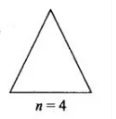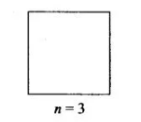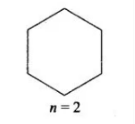Deepak Scored 45->99%ile with Bounce Back Crack Course. You can do it too!

# A uniform conducting wire of length 12a and resistance

Question:

A uniform conducting wire of length 12a and resistance R is wound up as a current-carrying coil in the shape of

(i) an equilateral triangle of side a;

(ii) a square if sides a and

(iii) a regular hexagon of sides a. The coil is connected to a voltage source V0. Find the magnetic moment of the coils in each case.

Solution:

(a) An equilateral triangle with side a

No.of loops = 4

Area of the triangle A = √3/4 a2

Magnetic moment, m = Ia2√3(b) For a square with sides a

Area, A = a2

No.of loops = 3

Magnetic moment, m = 3Ia2(c) For a regular hexagon with sides a

Area, A = 6√3/4 a2

No.of loops = 2

Magnetic moment, m = 3√3a2I